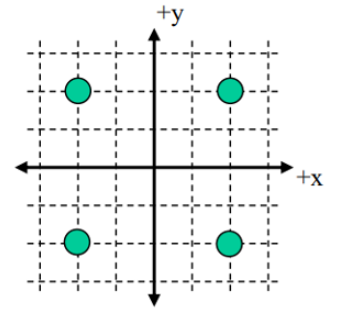# Problem: Four charges are situated as shown in the diagram (each grid line is separated by 1 meter). The two upper charges have a charge of +2 μC (+2×10-6 C). The two lower charges have a charge of-2 μC (-2x10-6 C).1. What is the magnitude of the net electric field at the point (0, 0)? a) 0 N/Cb) 2,250 N/Cc) 3,182 N/Cd) 6,364 N/Ce) 9,000 N/C 2) What is the net electric potential at the point (0, 2) on the positive y-axis? a) -19,900 Vb) -9,950 vc) 0 Vd) 9,950 Ve) 19,900 V 15) How much work would it take to bring a 5th charge of +2 μC from very far away to the origin? a) 0 Jb) 0.036 Jc) 0.144 Jd) 18,000 Je) 72,000J

###### FREE Expert Solution

1.

Electric field:

$\overline{){\mathbf{E}}{\mathbf{=}}\frac{\mathbf{k}\mathbf{q}}{{\mathbf{r}}^{\mathbf{2}}}}$

95% (39 ratings)###### Problem Details

Four charges are situated as shown in the diagram (each grid line is separated by 1 meter). The two upper charges have a charge of +2 μC (+2×10-6 C). The two lower charges have a charge of-2 μC (-2x10-6 C).1. What is the magnitude of the net electric field at the point (0, 0)?

a) 0 N/C
b) 2,250 N/C
c) 3,182 N/C
d) 6,364 N/C
e) 9,000 N/C

2) What is the net electric potential at the point (0, 2) on the positive y-axis?

a) -19,900 V
b) -9,950 v
c) 0 V
d) 9,950 V
e) 19,900 V

15) How much work would it take to bring a 5th charge of +2 μC from very far away to the origin?

a) 0 J
b) 0.036 J
c) 0.144 J
d) 18,000 J
e) 72,000J

Frequently Asked Questions

What scientific concept do you need to know in order to solve this problem?

Our tutors have indicated that to solve this problem you will need to apply the Electric Field concept. You can view video lessons to learn Electric Field. Or if you need more Electric Field practice, you can also practice Electric Field practice problems.